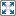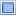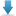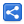# TypeScript - Using --noImplicitThis flag

[Last Updated: Mar 29, 2019]

## Wrong use of 'this' in functions

```class Rectangle {
constructor(w, h) {
this.w = w;
this.h = h;
}
getAreaFunction() {
return function() {
return this.w * this.h;
};
}
}
let rectangle = new Rectangle(2, 5);
let areaFunction = rectangle.getAreaFunction();
let area = areaFunction();
console.log(area);```

In above example, when areaFunction is called, 'this' does not refers to the enclosing Rectangle instance. In fact every standalone function defines its own 'this'. The runtime value of 'this' also depends on how function is called (via an object instance e.g. obj.func() or directly func()). In strict mode the value of 'this' is undefined if the function is called directly (func()).

### Use of --noImplicitThis flag in TypeScript

TypeScript can warn us about wrong use of 'this' if --noImplicitThis is used. Let's write above example in TypeScript:

#### this-wrong-use.ts

```class Rectangle{
private w: number;
private h: number;

constructor(w: number, h: number) {
this.w = w;
this.h = h;
}

getAreaFunction(){
return function():number{
return this.w * this.h;
}
}
}

let rectangle = new Rectangle(2,5);
let areaFunction = rectangle.getAreaFunction();
let area = areaFunction();
console.log(area);```

Let's compile above using --noImplicitThis flag:

```\$ tsc --noImplicitThis this-wrong-use.ts
this-wrong-use.ts:12:19 - error TS2683: 'this' implicitly has type 'any' because it does not have a type annotation.

12            return this.w * this.h;
~~~~

this-wrong-use.ts:12:28 - error TS2683: 'this' implicitly has type 'any' because it does not have a type annotation.

12            return this.w * this.h;
~~~~
```

## Fixing the problem

To fix above example we can use ES6 arrow function. An arrow function does not have its own 'this' but 'this' value of the enclosing execution context is used.

```class Rectangle{
private w;
private h;

constructor(w, h) {
this.w = w;
this.h = h;
}

getAreaFunction(){
return () => {
return this.w * this.h;
}
}
}

let rectangle = new Rectangle(2,5);
let areaFunction = rectangle.getAreaFunction();
let area = areaFunction();
console.log(area)```

`10`

## Example Project

Dependencies and Technologies Used:

• TypeScript 3.0.3
 Using --noImplicitThis flagSelect AllDownload• arrow-function-and-this
• arrow-function-and-this.ts

 Share